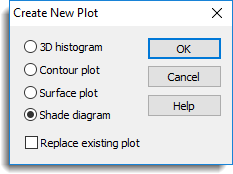1. Home
2. Create New Plot

# Create New Plot

Use this to redraw the current plot automatically as another type of plot. For example, if the current graph is a surface plot then you can redraw this as a contour plot without using the menus or commands in Genstat. You can create new plots using the same data set for the following graph types:

• 3D histogram
• Surface plot
• Contour plot